# Spf (E*[[x]]): Your walk through a flower garden

Inspired by the extraordinary expository style of Dr. Kazuya Kato, I’ve started reading parts of a (translated) Japanese children’s book when I’m stuck on a tough paper or concept — revisiting the concept with such a dreamlike world in mind usually unfolds an illustrative perspective. A misty world which begs to be put into firm ground via prolonged formal and concrete afterthought.

He embraces that teaching can be poetic and tantalizing, providing not a definition but a deep and creative hint that causes an exploratory shift in perspective, allowing you to walk down the path to the conclusion yourself. I wanted to try to exposit with this philosophy: confusion is expected and encouraged as impetus for reaching understanding. With that in mind, step into your flower garden.

Planted in a line of earth ($$\text{Spec }R$$) there are flowers, $$C$$, whose heads are smooth projective genus 1 curves with stems that can retract into the ground, s.t. the flower meets the earth at one point (a marked point).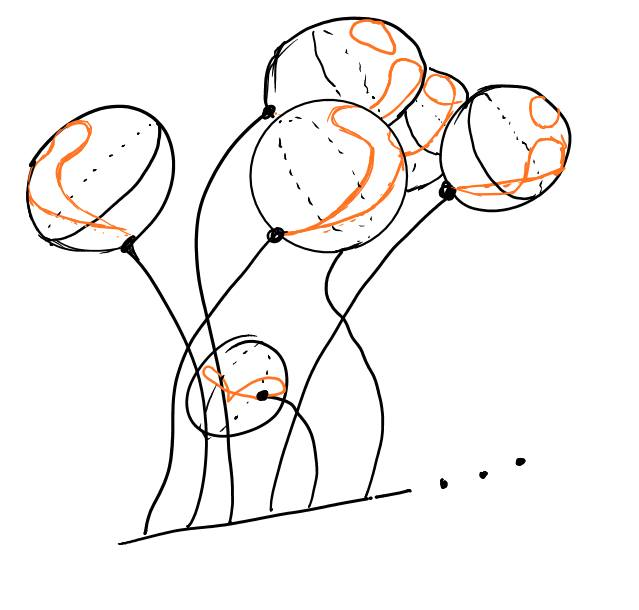Cutting the flowers off at their stems $$C \xrightarrow{p} \text{Spec }R$$ $$\Rightarrow$$ you’re left with the line of earth ($$\text{Spec }R$$)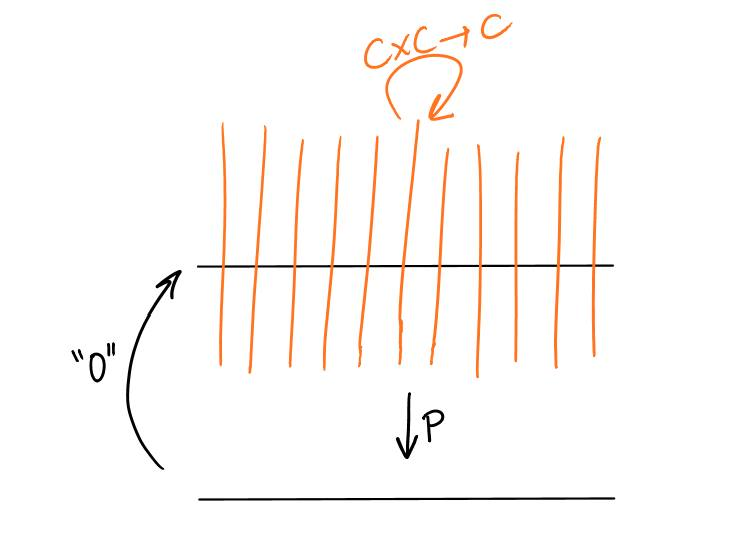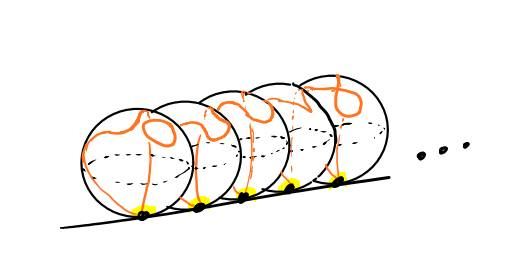Cutting into the petals a small ring around their stems (formal disk at marked point)

$$\Rightarrow$$ you’re left with the remaining (infinitely-layered) base of the flower sitting on top of $$\text{Spec }R$$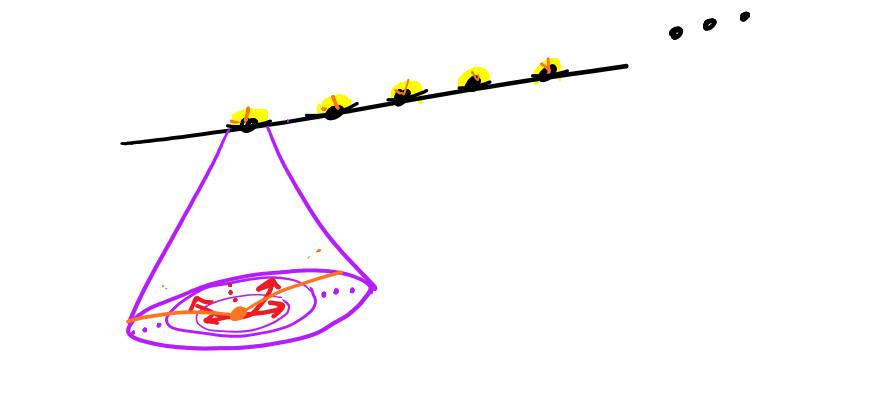Feeling frustrated that you can’t see clearly, you use your hands to move each disk lying flat on the ground to lay on its side, s.t. these bases are now stacked on top of each other like CDs and form a loose layered cylinder, centered around the line of earth.

1st layer = $$\text{Spec }R[x]/x^2$$, first infinitesimal neighborhood 1st&2nd layer = $$\text{Spec }R[x]/x^3$$, second infinitesimal neighborhood;…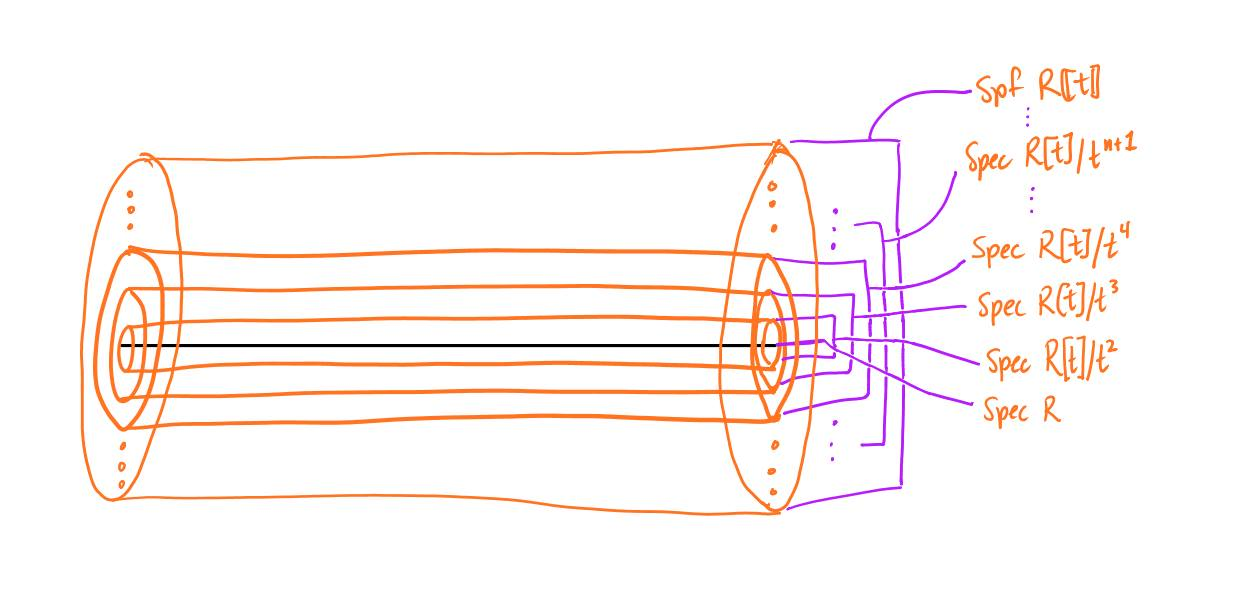You stare at this line of earth, adorned with (infinitely-layered) flower bases on top forming a layered tube around the line of earth. Looking closer, you see how the layers fit together, $$\text{Spf }R[[x]]$$.

(If you’d cut out the disk and forgotten how the layers fit together, you’d find yourself with $$\text{Spec }R[[x]]$$ — an awfully boring topology.)

Glance away, toward a different line of earth $$\text{Spec }E^$$ with (infinitely-layered) flower bases on top, $$CP^\infty_E := \text{Spf }E^(CP^\infty)$$.

Someone already cut the flowers down to their bases, before you had a chance to see them!

Flustered, you remember that $$CP^\infty$$ is the colimit of $$CP^n$$.

You reach into your pocket for your book-keeping device, and use it to look at the connectivity rings of each $$CP^n$$.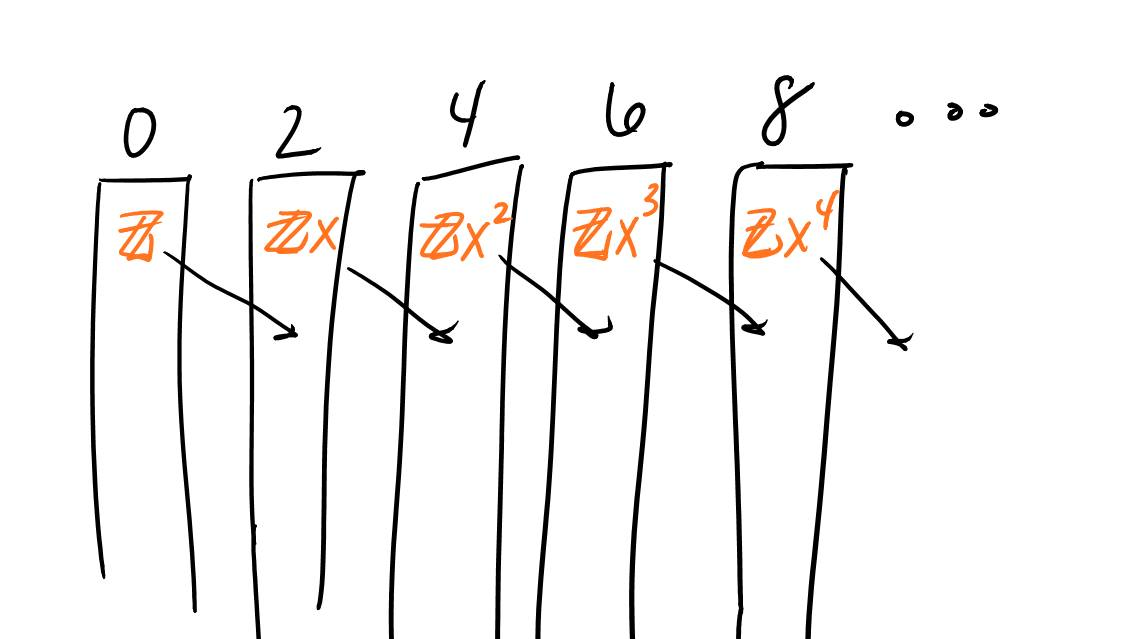Content, you label the layers of the flower base:

1st layer is Spec $$E^(CP^1)$$ = Spec $$E^[x]/x^2$$ 1st&2nd layer is Spec $$E^(CP^2)$$ = Spec $$E^[x]/x^3$$, …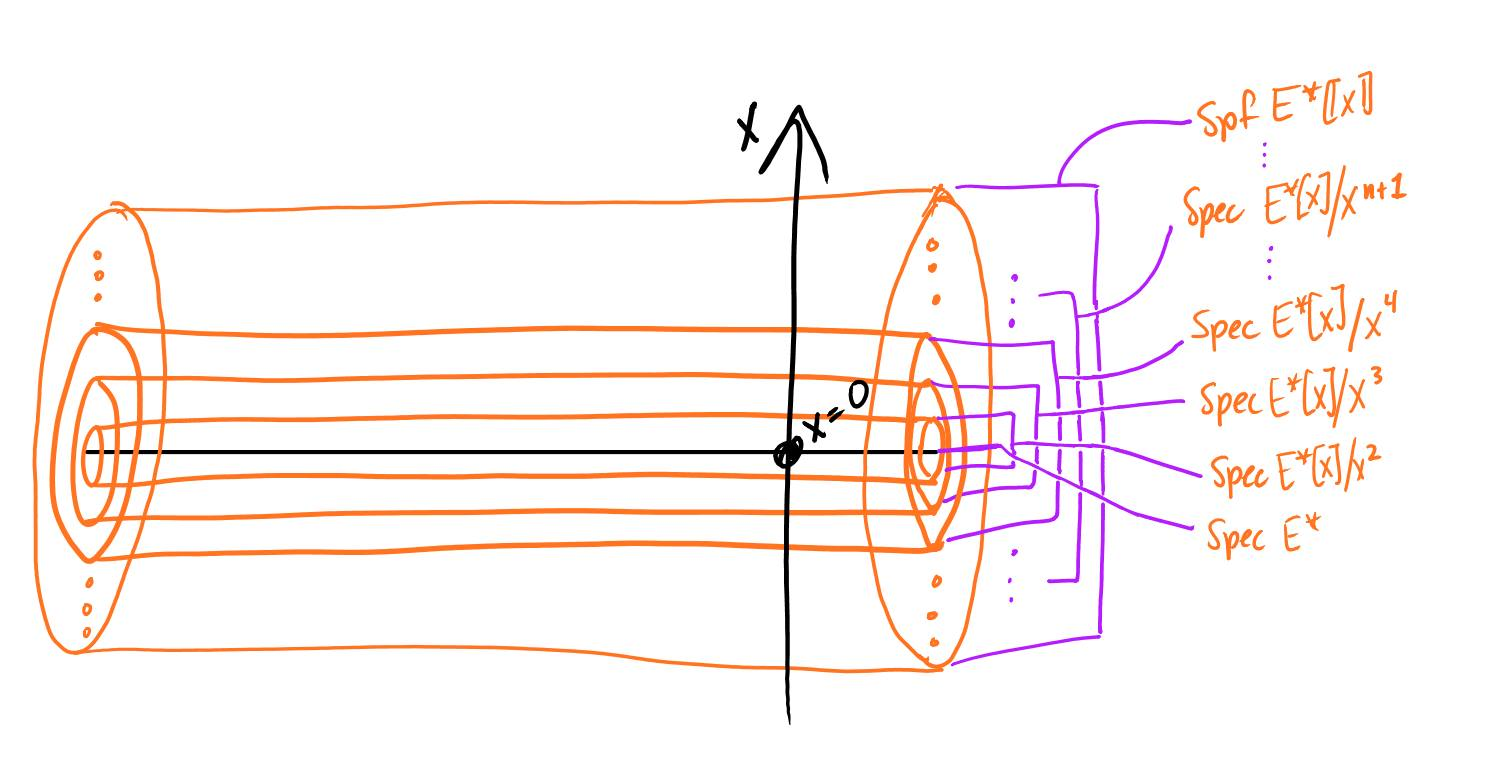Given the flower bases ($$CP^\infty_E$$), can you tell what flowers were over $$\text{Spec }E^*$$?

That is, is there a group object with a map to $$\text{Spec }E^*$$ whose formal completion along its 0-section is $$CP^\infty_E$$?

I thought the answer was no, but I think the answer is instead tautological. There is a H-space flower which we can trim to $$CP^\infty_E$$. What is it?

The notation for $$CP^\infty_E$$ itself is extremely suggestive.

The H-space in question is $$CP^\infty$$, and we’ve $$E$$-localized it!

You have enough to precisely decipher this story, each of your loose ends can be tied.

Written on May 27, 2015